Symmetric derivative

(diff) ← Older revision | Latest revision (diff) | Newer revision → (diff)

A generalization of the concept of derivative to the case of set functionson an-dimensional Euclidean space. The symmetric derivative at a pointis the limitwhere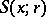is the closed ball with centreand radius, if this limit exists. The symmetric derivative of orderat a pointof a functionof a real variable is defined as the limit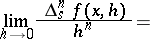A functionof a real variable has a symmetric derivative of order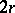at a point,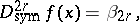if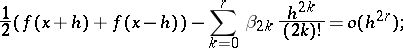and one of order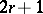,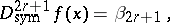if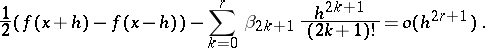Ifhas an-th order derivative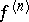at a point, then there is (in both cases) a symmetric derivative at, and it is equal to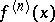.

How to Cite This Entry:
Symmetric derivative. Encyclopedia of Mathematics. URL: http://encyclopediaofmath.org/index.php?title=Symmetric_derivative&oldid=15476
This article was adapted from an original article by T.P. Lukashenko (originator), which appeared in Encyclopedia of Mathematics - ISBN 1402006098. See original article